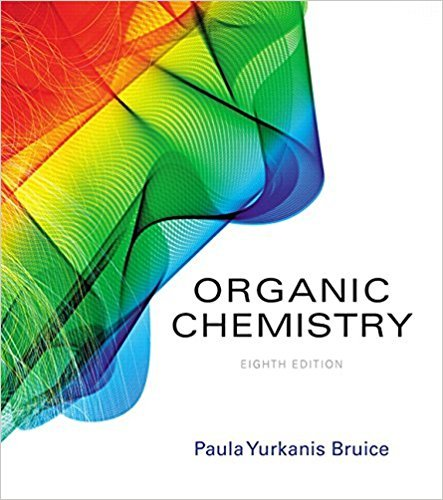×
×

# Solutions for Chapter 4.2: Using the E,Z System to Distinguish Isomers## Full solutions for Organic Chemistry | 8th Edition

ISBN: 9780134042282Solutions for Chapter 4.2: Using the E,Z System to Distinguish Isomers

Solutions for Chapter 4.2
4 5 0 234 Reviews
26
2
##### ISBN: 9780134042282

This textbook survival guide was created for the textbook: Organic Chemistry, edition: 8. Since 6 problems in chapter 4.2: Using the E,Z System to Distinguish Isomers have been answered, more than 31052 students have viewed full step-by-step solutions from this chapter. Organic Chemistry was written by and is associated to the ISBN: 9780134042282. Chapter 4.2: Using the E,Z System to Distinguish Isomers includes 6 full step-by-step solutions. This expansive textbook survival guide covers the following chapters and their solutions.

Key Chemistry Terms and definitions covered in this textbook
• alkali metals.

The Group 1A elements (Li, Na, K, Rb, Cs, and Fr). (2.4)

• amine

A compound that has the general formula R3N, where R may be H or a hydrocarbon group. (Section 16.7)

• bond order

The number of bonding electron pairs shared between two atoms, minus the number of antibonding electron pairs: bond order = (number of bonding electrons - number of antibonding electrons)/2. (Section 9.7)

• Brønsted–Lowry base

A substance (molecule or ion) that acts as a proton acceptor. (Section 16.2)

• Clemmensen reduction

Reduction of the C"O group of an aldehyde or ketone to a CH2 group using Zn(Hg) and HCl

• complete ionic equation

A chemical equation in which dissolved strong electrolytes (such as dissolved ionic compounds) are written as separate ions. (Section 4.2)

• diborane

B2H6. A dimeric structure formed when one borane molecule reacts with another.

• disulfide

A compound with the structure R!S!S!R.

A method for selectively cleaving and identifying the N-terminal amino acid of a polypeptide chain.

• endothermic

Any process with a positive DH (the system receives energy from the surroundings).

• ether

A compound in which two hydrocarbon groups are bonded to one oxygen. (Section 24.4)

• exothermic

Any process with a negative DH (the system gives energy to the surroundings).

• Furanose

A fi ve-membered cyclic form of a monosaccharide.

• gray (Gy)

The SI unit for radiation dose

• internal energy

The total energy possessed by a system. When a system undergoes a change, the change in internal energy, ?E, is defined as the heat, q, added to the system, plus the work, w, done on the system by its surroundings: ?E = q + w. (Section 5.2)

• Quaternary structure

The arrangement of polypeptide monomers into a noncovalently bonded aggregate.

• solute

A substance dissolved in a solvent to form a solution; it is normally the component of a solution present in the smaller amount. (Section 4.1)

• Solvolysis

A nucleophilic substitution in which the solvent is also the nucleophile

• staggered conformation

A conformation in which nearby groups in a Newman projection have a dihedral angle of 60°.

• Wolff-Kishner reduction

Reduction of the C"O group of an aldehyde or ketone to a CH2 group using hydrazine and a base. Ylide (Section 16.6)

×